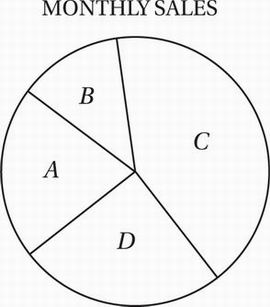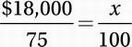# SAT Math Multiple Choice Question 730: Answer and Explanation

### Test Information

Question: 730

10.The pie graph above represents the monthly ad sales for four salespeople-Maria, Eli, Georgia, and Zoe-at a social media website. For the month, Maria's sales accounted for 25% of the total, Eli had \$3,000 in sales, Georgia had \$5,000 in sales, and Zoe had \$10,000 in sales.

What is the sum of the monthly sales for all four salespeople?

• A. \$22,500
• B. \$24,000
• C. \$25,000
• D. \$27,000

Explanation:

B

Data Analysis (pie graph) MEDIUM

Perhaps the simplest way to approach this is to notice that, since Maria's sales account for 25% of the total, the other salespeople must account for 100% - 25% = 75% of the total. Since this total is \$3,000 + \$5,000 + \$10,000 = \$18,000, we can find the total with a proportion.Cross multiply:

\$1,800,000 = 75x

Divide by 75:

\$24,000 = x